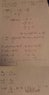# Solving LC-Circuit Problem: Q & I as Func. of Time

• gralla55

## Homework Statement

In an LC-circuit, with:

L = 64 mH
C = 121 nF

where at t=0,

Q = 10*10^-6 C , (the charge on the capasitor)
I = 0,3 A, (the current through the inductor)

Write an expression for the charge on the capacitor and the current through the inductor as a function of time.

## The Attempt at a Solution

I've tried this several times from stratch now, and while I do get an answer, according to the book I'm wrong. The standard form of a solution for Q, is Q = Qm*cos(wt + phi), where phi is the phase displacement. My strategy is to write both the expressions for Q and I, both containing the unknown parameters phi and Qm, and solving the system of two equations with two unknowns.

However, I always get phi = -1,20, whereas the answer in the book is phi = -0,922. I've attached my latest handwritten attempt at a solution, thanks for any help!

#### Attachments

•272.jpg
15.7 KB · Views: 401
Update:

I decided to check the answer in the book. The answer the book gives for the current function is:

I = 0,642*cos(Wt + pi/2 - 0,922), where w = 1,14*10^4

However, when setting t=0, this function gives an output I of 0,511. That is not equal to the problem's initial condition of I = 0,3!

The answer I got after substituting the variables back is:

I = 0,322*cos(wt + pi/2 - 1,2), where w = 11364

which coincidentally checks out as I = 0,3 when t=0.

I therefore conclude that the book is wrong, but I still would very much like a second opinion on this one. This is my first LC-circuit problem, so it's really hard to be too confident in my calculations.

Would greatly appreciate any help! :)

Your differential equation is wrong. The solution of LQ"-Q/C=0 is not periodic at all. There should be "+" instead of "-".

ehild

Try solving it directly using differential equation rather than using the derived formula. It is always easier and better to understand and solve the equations rather than memorizing formulae.

This will help. http://en.wikipedia.org/wiki/LC_circuit

You are correct, but it's just a typo, I solved it as if it were a plus. Anyway, I do know how to solve this differential equation. What I thought gave me trouble was solving for the initial conditions, but seeing how the answer in the book didn't check out when I tested it, whereas mine did, I actually do believe I got the right answer from the beginning.